### IMO Shortlist 1965 problem 5

Kvaliteta:
Avg: 0,0
Težina:
Avg: 0,0
Consider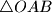$\triangle OAB$ with acute angle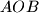$AOB$. Thorugh a point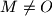$M \neq O$ perpendiculars are drawn to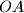$OA$ and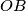$OB$, the feet of which are$P$ and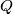$Q$ respectively. The point of intersection of the altitudes of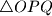$\triangle OPQ$ is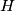$H$. What is the locus of$H$ if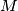$M$ is permitted to range over

a) the side$AB$;

b) the interior of$\triangle OAB$.
Izvor: Međunarodna matematička olimpijada, shortlist 1965